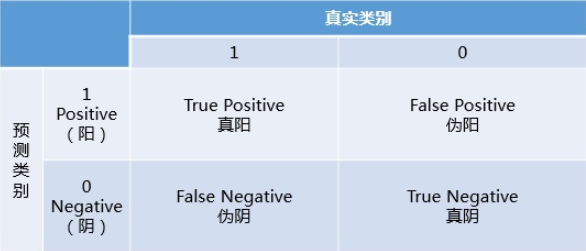2019/01/01

# 分类

## $\text{F}_{\beta}$

precision与recall是一对矛盾的度量。常常一个比较高时另一个就比较低，因此单一的precision或recall不能准确地衡量分类器的性能。 $F_{\beta}$通过权重系数$\beta$(表示recall的重要性是precision $\beta$倍)综合考虑precision与recall两个指标。当$\beta > 1$时，recall更重要；当$% $时，precision更重要。具体计算公式如下：

## ROC

• 预测类别为1的是Positive(阳性)，预测类别为0的是Negative(阴性)
• 预测正确用True(真)表示，预测错误用False(伪)表示• $TPR=\frac{TP}{TP+FN}$

• $FPR=\frac{FP}{FP+TN}$

• $TPR$(真阳率)表示在所有真实类别为1的样本中，预测类别为1的比例
• $FPR$(伪阳率)表示在所有真实类别为0的样本中，预测类别为1的比例

ROC以$FPR$为横轴，以$TPR$为纵轴。当两者相等时，表示无论真实类别是1还是0的样本，分类器将其预测为1的概率是相等的，即分类器对正例和负例样本毫无区分能力。因此一般来说$AUC$的最小值为0.5。

• 对于预测类别为离散标签，经统计得到混淆矩阵，从而算出一组$TPR$$FPR$，描点求面积可得AUC
• 对于预测类别为概率的分类器，依此使用所有的预测值作为阀值，根据得到的不同的混淆矩阵计算出一系列$TPR$$FPR$，描点求面积可得AUC

AUC的本质： 从所有正例中随机选取一个正样本A，再从所有负例中随机选取一个负样本B，分类器将A预测为正例的概率比将B预测为正例的概率还要大的可能性。AUC反映了分类器对样本的排序能力，即将正样本排在负样本前面的概率就是AUC的值。

## PR

PR(Precision - Recall)曲线以Precision为纵坐标，以Recall(TPR)为横坐标。与ROC一样，PR曲线也可以使用AUC来衡量分类器的效果。但ROC综合考虑了正负样本的分类情况，而PR曲线的两个指标都聚焦于正例。在类别不平衡问题中，由于主要关心正例，因此在此情况下PR曲线的效果要优于ROC曲线。